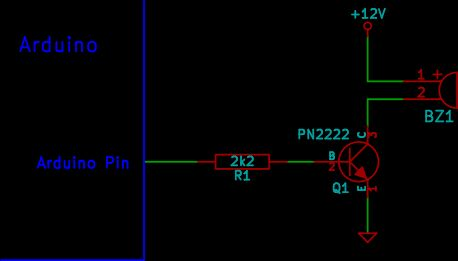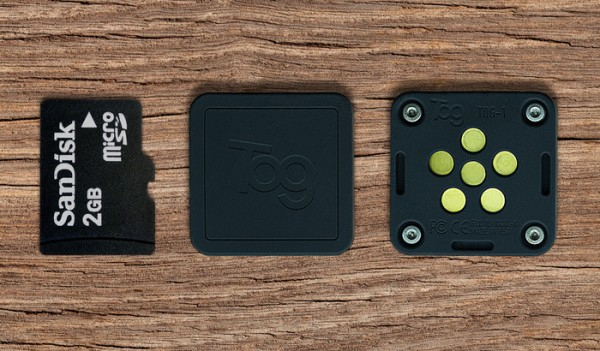# Arduino Buzzer Circuit

This article and circuit diagram show how to connect a buzzer to an Arduino when the buzzer operates at a different voltage to the Arduino. The buzzer may operate at 9V, 12V or some other voltage. Arduinos such as the Arduino Uno operate from 5V. All the voltages referred to here are d.c. voltages (direct current).
The same circuit used here can also be used to operate a 5V buzzer when the buzzer draws more current than the Arduino pin can handle, so can not be connected directly to the Arduino pin. To directly connect a low power 5V buzzer to an Arduino, refer to the article: Connecting a Buzzer to an Arduino Uno.## Arduino Buzzer Circuit Diagram

The circuit shown here uses a NPN transistor to connect the buzzer to the Arduino. The transistor allows the buzzer to be powered from a different voltage to the Arduino. Any NPN transistor that can handle the current drawn by the buzzer can be used.
With a PN2222 and 2k2 base resistor, the circuit can be used to operate a buzzer that draws up to about 200mA.

Although the circuit shows the buzzer being powered from 12V, the circuit allows any buzzer that operates from about 5V to around 24V to be used.
When the external power supply (12V in the circuit diagram) is connected, the GND or 0V of the power supply must be connected to the GND of the Arduino.
So the above circuit means that the connections must be made as follows:
The power supply powering the buzzer in this circuit is a 12V battery.## Arduino Sketch

The Arduino sketch below operates the above circuit by pulsing the buzzer on for a short period of time continuously.
This video shows the buzzer powered from a 9V battery and the Arduino powered from a USB cable. The Arduino is running the above sketch.

For more detail: Arduino Buzzer Circuit

#### This Post / Project can also be found using search terms:

• arduino buzzer circuit
• how to create an active buzzer circuit with an arduino mega 2560 board code
• arduino based buzzer circuit with arduino as isp
• arduino circiut with buzzer